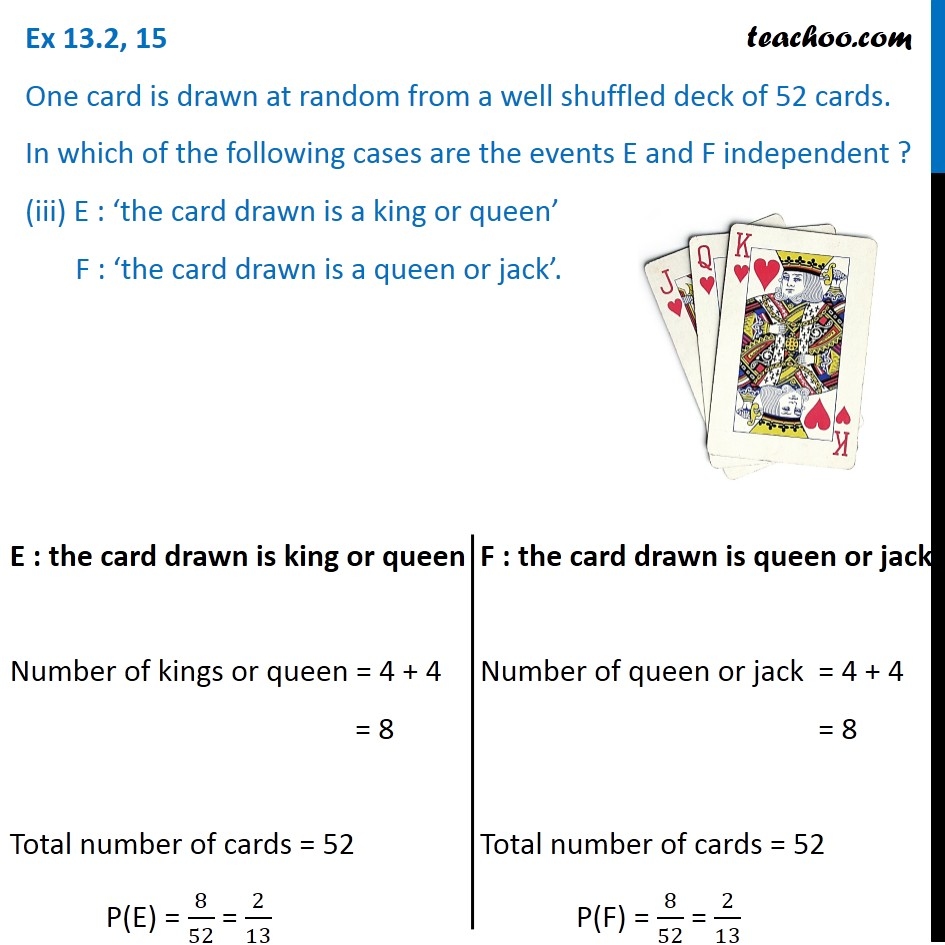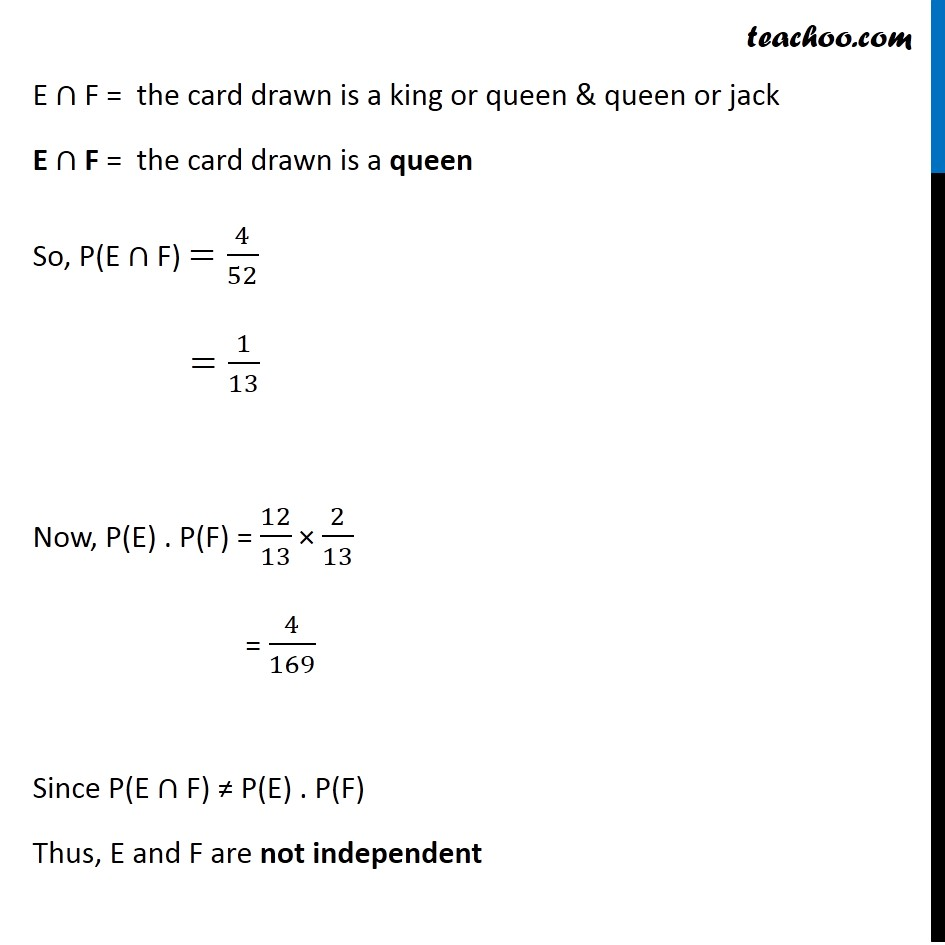Ex 13.2

Chapter 13 Class 12 Probability
Serial order wiseLearn in your speed, with individual attention - Teachoo Maths 1-on-1 Class

### Transcript

Ex 13.2, 15 One card is drawn at random from a well shuffled deck of 52 cards. In which of the following cases are the events E and F independent ? (iii) E : ‘the card drawn is a king or queen’ F : ‘the card drawn is a queen or jack’.Finding P(E), P(F) and P(E ∩ F) E : the card drawn is king or queen Number of kings or queen = 4 + 4 = 8 Total number of cards = 52 P(E) = 8/52 = 2/13 F : the card drawn is queen or jack Number of queen or jack = 4 + 4 = 8 Total number of cards = 52 P(F) = 8/52 = 2/13 Now, E ∩ F = the card drawn is a king or queen & queen or jack E ∩ F = the card drawn is a queen So, P(E ∩ F) =4/52=1/13 Now, P(E) . P(F) = 12/13 × 2/13 = 4/169 ≠ P(E ∩ F) Since P(E ∩ F) ≠ "P(E) . P(F)" Thus, E and F are not independent